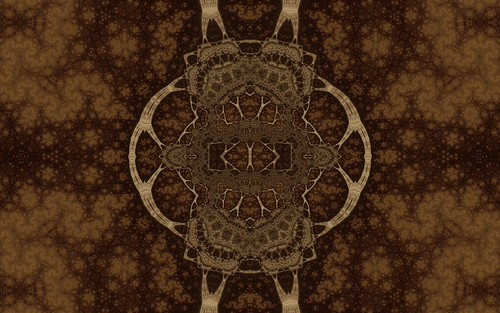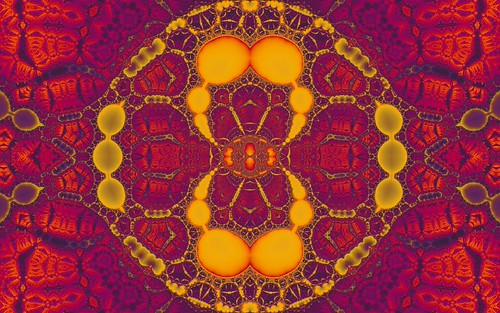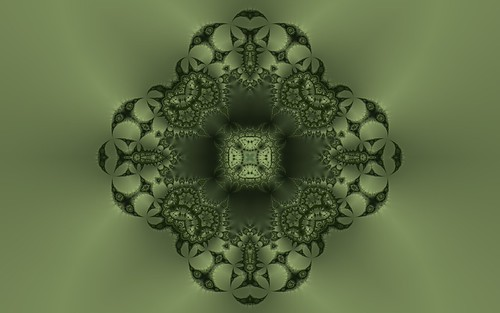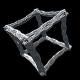# Kalisets and Hybrid Ducks

I saw a new Ducks fractal variation named Kalisets in this fractalforums post by Kali.

The new variation uses the formula z=abs(z^-1)+c. It turns out this is actually the burning ship formula with power -1 rather than power -2. Like the Ducks fractals the “Mandelbrot” variety (c is set to the value of each pixel prior to iterating) of this formula gives rather boring images, but the “Julia” variety (c is constant across the image) leads to new interesting results.After trying different variaitons of the formula I came up with these three.

z=log(abs(z^-1)+c)z=log(sin(abs(z^-1))+c)z=sin(abs(z^-1))+cUsing the hybrid fractal principal also works fine with these formulas. For the hybrid fractals the formula, variation and power are alternated between iterations.Higher resolution images are avilable in my flickr Ducks set.

The most interesting thing about these new types of fractals is how the Mandelbrot versions are boring but the Julia versions give rise to these complex patterns and structures. People must have tried these formulas in the past but possibly once they saw the boring Mandelbrot version they gave up and tried other formulas. There are bound to be many more formulas based on these principals found in the future.

The above new variations are now included in the latest version of Visions Of Chaos.

Jason.

## 3 responses to “Kalisets and Hybrid Ducks”

1.Dan Wills says:

Spot on Jason, some awesome new work here!

I’ve explored some of these types of fractals too but generally only looked at the mandelbrot variant. For the spacefilling formulae I already knew, I found the julia var less interesting but it’s good to know that it’s worth checking out the julia var when exploring a new formula.

This and Kali’s work are brilliant, and so are Sam Monnier’s coloring insights. It’s a very interesting time for space-filling fractals!

2.Iain Poole says:

What is the actual definition of a “hybrid” fractal? Are there any textbooks or guidance notes on this?

•softologyblog says:

Hybrid means you iterate the fractal by alternating different formula types.

ie, your first iteration may use the original ducks formula, and then switch to the z=log(abs(z^-1)+c) for one iteration and then back to the original. This repeats for the number of usual fractal iterations each pixel.

You can have more than 2 formulas too or change the iteration counts for each formula or have different powers for each formula used. Any combo to mix up and combine formulas.

I originally saw the idea on Fractal Forums a long while back. Not sure if there is any official textbook definition or explanation anywhere else.